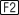Module 1 - Describing Functions Introduction | Lesson 1 | Lesson 2 | Lesson 3 | Lesson 4 | Self-Test Self Test What is the Rule of Three? What is the Home screen? Define the function g(x) = 2x3 – x2 – 8x + 4 and use theAlgebra solve( command to find the roots. Graph g(x). Use the following window settings: xmin = -5 xmax = 5 xscl = 1 ymin = -5 ymax = 10 yscl = 1 xres = 2 Use the GraphMath Zero feature to find the roots of g(x). Make a table of values for g(x). Let x start at -3 and increase by 1. Use table zoom to approximate the root between 0 and 1. Click here to check your answers. < Back ©Copyright 2007 All rights reserved. | Trademarks | Privacy Policy | Link Policy